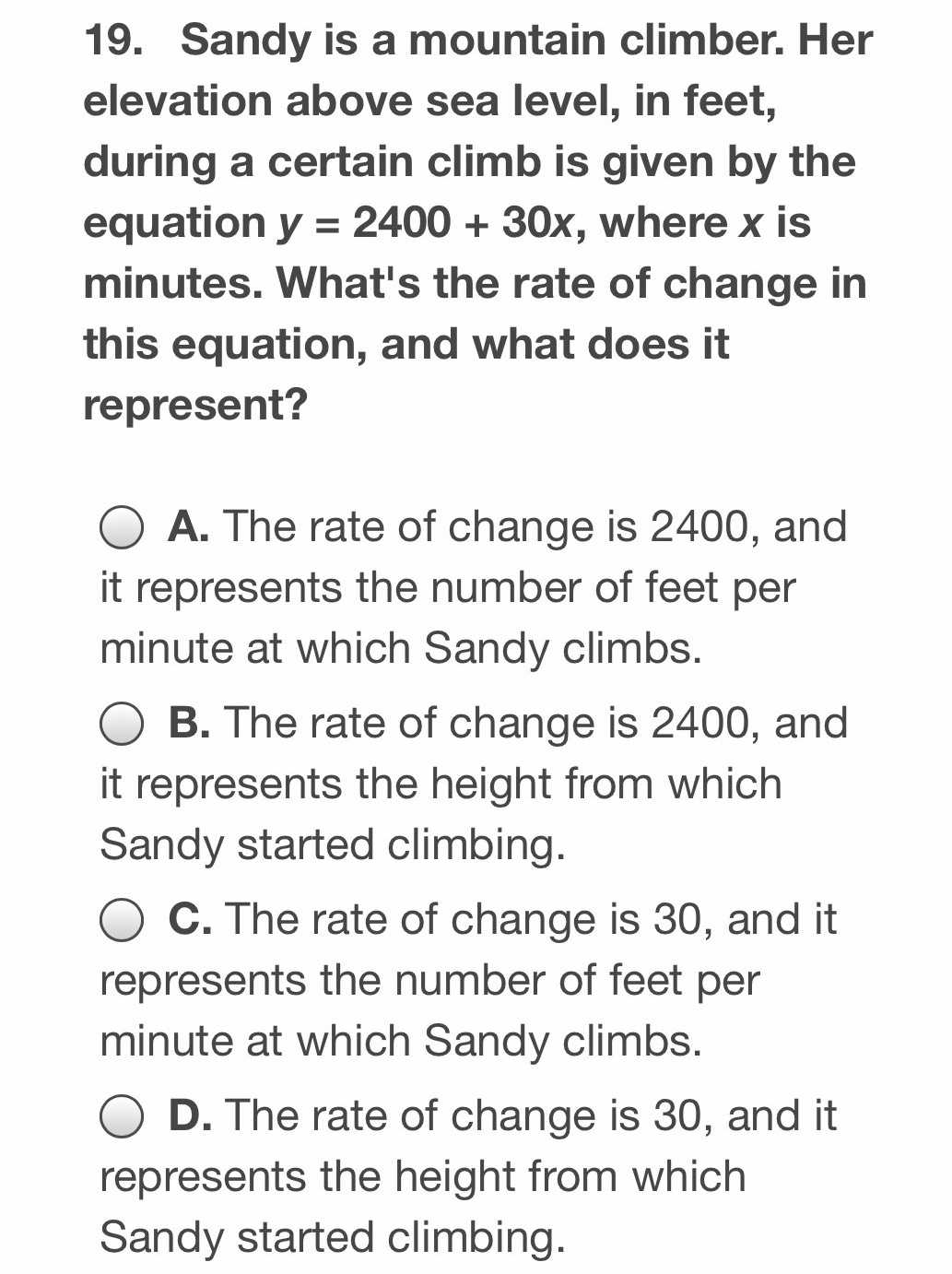### ¿Todavía tienes preguntas de matemáticas?

Pregunte a nuestros tutores expertos
Algebra
Pregunta19. Sandy is a mountain climber. Her elevation above sea level, in feet, during a certain climb is given by the equation $$y = 2400 + 30 x ,$$ where $$x$$ is minutes. What's the rate of change in this equation, and what does it represent?

A. The rate of change is $$2400$$ , and it represents the number of feet per minute at which Sandy climbs.

B. The rate of change is $$2400$$ , and it represents the height from which Sandy started climbing.

C. The rate of change is $$30$$ , and it represents the number of feet per minute at which Sandy climbs. D. The rate of change is $$30$$ , and it represents the height from which Sandy started climbing.